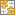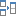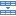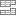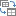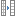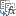# How to do calculations in Excel

The tutorial shows how to do arithmetic calculations in Excel and change the order of operations in your formulas.

When it comes to calculations, there is almost noting that Microsoft Excel cannot do, from totaling a column of numbers to solving complex linear programming problems. For this, Excel provides a few hundred predefined formulas, called Excel functions. In addition, you can use Excel as a calculator to do math - add, divide, multiply, and subtract numbers as well as raise to power and find roots.

## How to do calculations in Excel

Making calculations in Excel is easy. Here's how:

• Type the equal symbol (=) in a cell. This tells Excel that you are entering a formula, not just numbers.
• Type the equation you want to calculate. For example, to add up 5 and 7, you type =5+7
• Press the Enter key to complete your calculation. Done!

Instead of entering numbers directly in your calculation formula, you can put them in separate cells, and then reference those cells in your formula, e.g. =A1+A2+A3

The following table shows how to perform basic arithmetic calculations in Excel.

 Operation Operator Example Description Addition + (plus sign) =A1+A2 Adds up the numbers in cells A1 and A2. Subtraction - (minus sign) =A1-A2 Subtracts the number in A2 from the number in A1. Multiplication * (asterisk) =A1*A2 Multiplies the numbers in A1 and A2. Division / (forward slash) =A1/A2 Divides the number in A1 by the number in A2. Percent % (percent) =A1*10% Finds 10% of the number in A1. Raising to power (Exponentiation) ^ (caret) =A2^3 Raises the number in A2 to the power of 3. Square root SQRT function =SQRT(A1) Finds the square root of the number in A1. Nth root ^(1/n) (Where n is the root to find) =A1^(1/3) Finds the cube root of the number in A1.

The results of the above Excel calculation formulas may look something similar to this: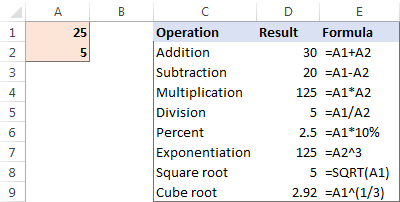Apart from that, you can combine values from two or more cells in a single cell by using the concatenation operator (&) like this:

`=A2&" "&B2&" "&C2`

A space character (" ") is concatenated in between cells to separate the words: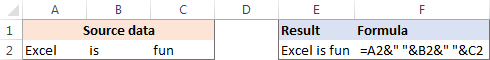You can also compare cells by using logical operators such as "greater than" (>), "less than" (<), "greater than or equal to" (>=), and "less than or equal to" (<=). The result of comparison are logical values of TRUE and FALSE: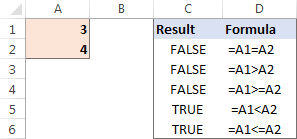## The order in which Excel calculations are performed

When you do two or more calculations in a single formula, Microsoft Excel calculates the formula from left to right, according to the order of operations shown in this table:

 Precedence Operation 1 Negation, i.e. reversing the number sign, as in -5, or -A1 2 Percent (%) 3 Exponentiation, i.e. raising to power (^) 4 Multiplication (*) and division (/), whichever comes first 5 Addition (+) and subtraction (-), whichever comes first 6 Concatenation (&) 7 Comparison (>, <, >=, <=, =)

Since the order of calculations affects the final result, you need to know how to change it.

## How to change the order of calculations in Excel

Like you do in math, you can change the order of Excel calculations by enclosing the part to be calculated first in parentheses.

For example, the calculation `=2*4+7` tells Excel to multiply 2 by 4, and then add 7 to the product. The result of this calculation is 15. By enclosing the addition operation in parentheses `=2*(4+7)`, you instruct Excel to add up 4 and 7 first, and then multiply the sum by 2. And the result of this calculation is 22.

Another example is finding a root in Excel. To get the square root of, say, 16, you can use either this formula:

`=SQRT(16)`

or an exponent of 1/2:

`=16^(1/2)`

Technically, the above equation tells Excel to raise 16 to the power of 1/2. But why do we enclose 1/2 in parentheses? Because if we don't, Excel will raise 16 to the power of 1 first (an exponent operation is performed before division), and then divide the result by 2. Since any number raised to the power of 1 is the number itself, we would end up dividing 16 by 2. In contrast, by enclosing 1/2 in parentheses you tell Excel to divide 1 by 2 first, and then raise 16 to the power of 0.5.

As you can see in the screenshot below, the same calculation with and without parentheses produces different results: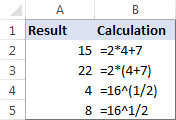This is how you make calculations in Excel. I thank you for reading and hope to see you on our blog next week!

### 39 responses to "How to do calculations in Excel"

1.Dave says:

Could you show us how to build a financial model in Excel?

2.Rae says:

Hi, I have a 2 part question.
1st, upon looking at my formula to try to reverse engineer it, I noticed the math seems a bit weird. I have this formula to calculate the amounts of 2 components based on the ratio and the total weight (tw=total weight, ra=ratio a, rb=ratio b, aw=weight of part a, bw=weight of part b. I'll use these instead of the cell #'s here). My formula looks like this bw=tw*(rb/(ra+rb)) and the formula for the other part is ra=tw*(ra/(ra+rb)).
the issue is that when I do out the math in steps, based on order of operations, I get a decimal, but the formula gives me a whole #. Why don't I get the same result?
Part 2; how can I create 2 formulas that will solve this backwards, as in, plug in the weight of a or b and get what my total weight and the weight of the other part as a result?
Thank you so much! I've been cracking my brain open over this for days and searching all over the web for an answer that I don't know an easy question to!!

•Rae says:

sorry, messed up the 2nd formula, that should be aw=tw*(ra/(ra+rb))

3.Rae says:

One last point, sorry to go on:
to illustrate, I'll plug in 66 as the tw, 100 as ra and 10 as rb. in the formula I get 60 and 6 for a and b, which seems like it did not respect the parenthesis, as 66*(100/(100+10))= 66*(100/110)= 66*0.9090909091= 60.0000000006, while 66*100/(100+10)=6600/110=60 which is what the formula gives me.

•Irina Goroshko (Ablebits.com Team) says:

Hi Rae,
1. Your formula is correct, you do not get the same result because of
rounding off. Check the result again with this formula (it will round to 10 decimal places):
=TEXT(66 * ROUND((100 / (100 + 10)),10),"0.0000000000")

2. Try these formulas:
tw= aw/(ra/(ra+rb))

or

= TEXT(60 / ROUND((100 / (100 + 10)),10),"0.0000000000")
tw=bw/(rb/(ra+rb))

or

= TEXT(6 / ROUND((10 / (100 + 10)),10),"0.0000000000")

And your last point, try the following:
bw=(aw/(ra/(ra+rb)))*(rb/(ra+rb))
aw=(bw/(rb/(ra+rb)))*(ra/(ra+rb)).

4.andrews says:

You are so good, I've learn a lot from you expecially the capitalization and the calculations.

good programmer keep it up

5.johanna says:

=(B50*C50)+(B51*C51)+(B52*C52)/2.378
How do I get all three items in brackets to total then divide by 2.378

•James says:

Formula is =((B50*C50)+(B51*C51)+(B52*C52))/2.378

6.jeni says:

Thanks a lot!!

7.Kinz says:

Hey! how do i divide a number into 10000, 5000, 1000 and 250? (For ex. I want to divide 77350 into 7(10000) 1(5000) 2(1000) 2(250) so it would also round up to 77500 (the numbers should end either by 000, 250, 500 or 750)

8.SOPHIA says:

Theory Hours Practical Hours Total Hours
Prescribed Attended % Prescribed Attended % Prescribed Attended %

150 99.00 150 100 300 99.50
150 97.00 500 100 650
150 95.00 100 100 250 95.50
150 87.00 900 100 1050 87.50
600 95% 1650 100% 2250

•Mary Trifuntova (Ablebits.com Team) says:

Hello, Sophia,
Thank you for contacting us.

9.Joe says:

Can I configure cells to automatically calculate pre-determined costs prior to entering the primary value? i.e. if I have a fixed shipping cost but the price of the product varies.

10.Ben says:

Can you advise how to set up excel to execute this formula.
13.6 x [(SL + SS) – W.T.] x W.T.
I'm trying to set up a spreadsheet for a user to input SL, SS and W.T. to calculate the weight of an item.

11.Suzy says:

Formula: Qty-QtySold-Qtyreturned

If I start with 4 -1-1 it will equal 2 This is Correct
If I start with 4 and leave blank the sold or returned fields the result shows BLANK.
I am so frustrated and cannot figure out what is wrong>>>> Please advise Thank you.

12.Yogesh tailor says:

Row A B C D
10 15 20 25 THIS FOR ROW VALUE 0.50 RS SUBTRACT ONE FORMULA
5 10 15 20 ONE LINE FORMULA

13.Ahmadshah says:

Great and the greatest work has done! I learned very easily Microsoft excel

14.hazim says:

hi how to key in this formula in excel =(10000×5266)-(10000×342)/(1.319)×(16841-11368/1.319)-1072.4)^2)*(100+6.0)*(1.458)
thanks。

15.Wondering says:

I am not the most skilled at excel , but am enjoying the discovery as i go.
In creating a calculating option for a tool i am working on, I am wanting the number 1 to calculate to total a maximum of 5 as 1 is selected from a drop down option.

Using conditional formatting and data validation i cannot get the the numbers to add when i want to determine a difference with the same number.

For example i have selected number 1 in the drop down list for selection along with other choices. The selection I want is listed below (as laid out in data validation.)
1, 1L, 1E,
I want 1 L and 1 E a certain color like green (using Conditional formatting) to pop up automatically as 1 L or 1 E is selected.
I want the number 1 alone to have no color
I want to have all the number 1'S to calculate as they are selected
1 L and 1 E dont calculate (1 and anything i put dont calculate, )
is there a way?? Can you help?
I hope this makes sense

16.Kerleisha says:

hi good day
while typing my stuff in excel and trying the calculate when I put a =B1+B2 the part with the B2 turns to read and the end product just says value can u all tell me where I went wrong

•Alexander Trifuntov (Ablebits.com Team) says:

Hello Kerleisha!
Probably, the error occurs because you have a number in one of the cells and the other cell contains a symbol, e.g. a space.
If it is not the case, please describe the problem in more detail so I will be able to help you better.

17.Ram says:

Excel alloys _______ calculation

18.Guled says:

No. Salary Range New Tax Rate. Deduction
1. 0 – 600 Birr. Non-Taxable –
2 601 – 1,650 Birr 10%. 60 Birr
3. 1,651 – 3,200 Birr. 15% 142.50 Birr
4. 3,201 – 5,250 Birr. 20% 302.50 Birr
5. 5,251 – 7,800 Birr. 25%. 565 Birr
6. 7,801 – 10,900 Birr 30%. 955 Birr
7. Over 10,900 Birr 35%. 1,500 Birr how do i make a formula

•Alexander Trifuntov (Ablebits.com Team) says:

Hello Guled!
For me to be able to help you better, please describe your task in more detail. It’ll help me understand it better and find a solution for you. Thank you.

19.Mark says:

Hi,
If I would like to multiply (10000 x 8%) ÷12. Is the formula (10000*8%)/12? I can't seem to get it right on excel. And also if I want to calculate (10000x8%) x 2 then ÷ 24, What will be the formula like? Appreciate your time and thank you in advance!

•James says:

formula would be =(10000*.08)/12

20.Matthew Gannon says:

Question.. I want to have this formula in a cell (200)/(1+x/100)=n, I want the formula to be invisible in the box and only put out a number (n) when I type in a value for x in the formula cell, thanks

•Alexander Trifuntov (Ablebits.com Team) says:

Hello Matthew!

=IF(A1 <> "",(200)/(1+A1/100),"")

The formula will show the value only if cell A1 is not empty

21.Mark Nikrandt says:

Hi, I need to calculate the Inductance formula. The Reactance (XL) is in cell K56 and f=60. The calculation is XL/2PIf. I have tried ((K56)/(2*pi*60)) and variations of this and cannot get it correct. Can you help?

22.Rhonda says:

Hello,
I have excel directories to track test conducted at each location. The directory has columns that state positive or negative with result testing. How do I create a summary page that will calculate the positive and negative findings?

Thank you,
Rhonda

•Alexander Trifuntov (Ablebits.com Team) says:

Hello!
Unfortunately, without seeing your data it hard to give you advice. I recommend you a guide to working with pivot tables. If you have more specific questions and can give an example of the initial data and the desired result, I will try to help you.

23.Calkvin says:

Excel spread sheet, why when making an percentage formular calculation does it show a negative amount?

•Alexander Trifuntov (Ablebits.com Team) says:

Hello!
If you count the percentage of a negative number, the result will also be a negative number.

24.Daria says:

Hello,

Total discount to distributor 42%, they have margin of 9%, what will be the % discount that end customer receives?

25.Charles says:

I have to calculate (11/6) to the power 54. How can I do this on excel? I cannot find the caret function on excel.

•Alexander Trifuntov (Ablebits.com Team) says:

Hello!

=(11/6)^54

The ^ character is at 6 using SHIFT

26.Robin says:

how do I use a formula on a spreadsheet on a number of entries without having to enter it each time

27.abubakar says:

thanks but seriously I don't get it all I need more explanation from the scratch

Ultimate Suite for Microsoft Excel